# SSAT Upper Level Math : How to find the length of the side of an equilateral triangle

## Example Questions

### Example Question #1 : Equilateral Triangles

An equilateral triangle has a perimeter of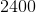units. What is the length of each side?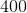units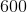unitsunits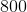unitsunits

Explanation:

Because an equilateral triangle has three sides that are the same length, divide the given perimeter by 3 to find the length of each side.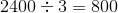### Example Question #2 : Equilateral Triangles

The perimeter of a equilateral triangle is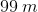. In meters, what is the length of a side of this triangle?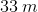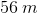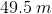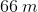Explanation:

Since all three sides are equal in an equilateral triangle, we can just divide the perimeter by 3 to find a side length.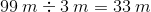### Example Question #1 : Equilateral Triangles

The perimeter of an equilateral triangle is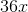. What is the length of a side of this triangle?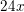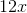Explanation:

Because all the sides in an equilateral triangle are equal, we can just divide the perimeter by 3 to find the length of a side.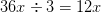### Example Question #4 : Equilateral Triangles

The perimeter of an equilateral triangle is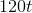. What is the length of one side of the triangle?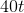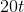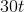Explanation:

Since an equilateral triangle has sides that are all the same, divide the perimeter byto get the length of each side.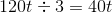### Example Question #5 : Equilateral Triangles

The perimeter of an equilateral triangle is 39 meters. In meters, how long is one side of the triangle?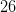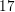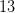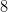Explanation:

An equilateral triangle has sides that are the same length. Then, to find the length of one side, you only need to divide the perimeter by 3.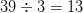### Example Question #6 : Equilateral Triangles

The perimeter of an equilateral triangle is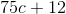. What is the length of one side of this triangle?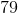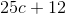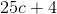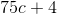Explanation:

Since an equilateral triangle has three equal sides, divide the perimeter byto find the length of each side.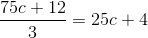### Example Question #7 : Equilateral Triangles

The perimeter of an equilateral triangle is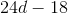. What is the length of one side of this triangle?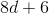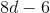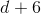Explanation:

Since an equilateral triangle has three equal sides, divide the perimeter byto find the length of one side.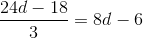### Example Question #1 : How To Find The Length Of The Side Of An Equilateral Triangle

What is the difference between an equilateral triangle and a scalene triangle?

The number of side lengths

The length of their sides

Their color

The sum of their angle measurements

The sum of their side lengths

Of the choices listed, the main difference between an equilateral triangle and a scalene triangle is their side lengths. An equilateral triangle has to haveequal sides, but a scalene triangle can have all different side lengths.